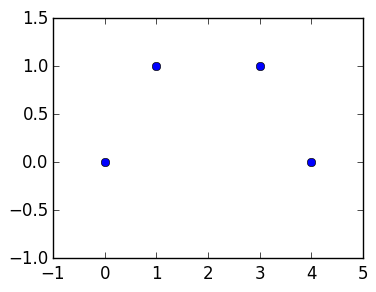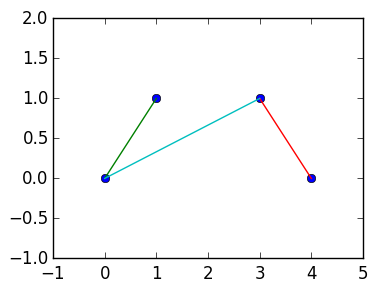# Lab 3: Structures¶

In this lab, we will investigate solving and plotting a 2D version of the spring system introduced in Lecture 6, where now the springs are replaced with bars that can be elongated but not bent, and the bars are attached at points to create structures. We will use linear algebra to understand which structures are stable: that is, won't fall down.

Remark You will not be examined on physics or modelling in this course. The physical systems studied are only to facilitate understanding the computational aspects of the problem.

## Structures as matrices of nodes and bars¶

We must first set up a way to interact. A structure consists of nodes and bars, where each bar is always attached to two nodes. We define the nodes by a sequence of $n$ points in 2D:

$$\mathbf{a}_1=(x_1,y_1),\mathbf{a}_2=(x_2,y_2),\ldots,\mathbf{a}_n=(x_n,y_n).$$

The bars are then given by a sequence of $m$ pairs of nodes:

$$\mathbf{b}_1=(p_1,q_1),\mathbf{b}_2=(p_2,p_2),\ldots,\mathbf{a}_n=(p_n,q_n),$$

where $p_j$ and $q_j$ are integers between 1 and $n$. For example, the bar attaching $\mathbf{a_2}$ to $\mathbf{a_4}$ is represented as $(1,4)$.

We will represent the nodes by a $2 \times m$ matrix

$$A=\begin{pmatrix} x_1 & x_2 & \cdots & x_n \cr y_1 & y_2 & \cdots & y_n \end{pmatrix}$$

and the bars by a $2 \times m$ matrix

$$B=\begin{pmatrix} p_1 & p_2 & \cdots & p_n \cr q_1 & q_2 & \cdots & q_n \end{pmatrix}$$

In Julia, the nodes $A$ will be represented by a Matrix{Float64}, for example, the following matrix represents nodes at $(0,0),(1,1),(3,1),(4,0)$:

In :
A=[0. 1. 3. 4.;
0. 1. 1. 0.]

n=size(A,2)  # number of nodes

Out:
4

Note that size(A,1) gives the number of rows of A and size(A,2) gives the number of columns of A.

Theb bars $B$ will be represented by a Matrix{Int}. For example, the following represents the bars connecting nodes 1 and 2, 2 and 3, and 3 and 4:

In :
B=[1 2 3;
2 3 4]

m=size(B,2)  # number of nodes

Out:
3

## Accessing and altering subsections of arrays¶

We will use the following notation to get at the columns and rows of matrices:

A[a:b,k]    # returns the a-th through b-th rows of the k-th column of A as a Vector of length (b-a+1)
A[k,a:b]    # returns the ath through bth columns of the k-th row of A as a 1 x (b-a+1) Matrix
A[:,k]      # returns all rows of the k-th column of A as a Vector of length size(A,1)
A[k,:]      # returns all columns of the k-th row of A as a 1 x size(A,2) Matrix
A[a:b,c:d]  # returns the a-th through b-th rows and c-th through d-th columns of A
# as a (b-a+1) x (d-c+1) Matrix


The ranges a:b and c:d can be replaced by any AbstractVector{Int}. For example:

A[[1,3,4],2]  # returns the 1st, 3rd and 4th rows of the 2nd column of A


The function vec turns a matrix into a vector:

vec(A[k,:])  # returns all columns of the k-th row of A as a size(A,2) Vector


Exercise 1 Can you guess what A[2,[1,3,4]] returns, using the definition of A as above? What about A[1:2,[1,3]]? And A[1,B[1:2,1]]? And vec(A[1,B[1:2,1]])?

In :
A[2,[1,3,4]],A[1:2,[1,3]],A[1,B[1:2,1]],vec(A[1,B[1:2,1]])

Out:
(
1x3 Array{Float64,2}:
0.0  1.0  0.0,

2x2 Array{Float64,2}:
0.0  3.0
0.0  1.0,

1x2 Array{Float64,2}:
0.0  1.0,

[0.0,1.0])

We can also use this notation to modify entries of the matrix. For example, we can set the 1:2 x 2:3 subblock of A to [1 2; 3 4] as follows:

In :
A[1:2,2:3]=[1 2; 3 4]
A

Out:
2x4 Array{Float64,2}:
0.0  1.0  2.0  4.0
0.0  3.0  4.0  0.0

Exercise 2 What do you think the following returns?

In :
A=[0. 1. 3. 4.;
0. 1. 1. 0.]
A[1:2,[1,3]]=[6 7; 8 9]
A

Out:
2x4 Array{Float64,2}:
6.0  1.0  7.0  4.0
8.0  1.0  9.0  0.0

## Creating a type to represent a structure¶

Rather than working with matrices directly, it is better to work with a special type to represent structures. This way we can incorporate special behaviour directly. We are going to implement routines for the following type:

In :
type Structure
nodes::Matrix{Float64}
bars::Matrix{Int64}
end


We want to be able to create a Structure just with nodes, and then add bars later. Last lecture we learned about constructors that verify arguments. But we can also create functions with the same name as a type: these are called external constructors. The following makes a structure with only nodes, and no bars:

In :
function Structure(nodes::Matrix{Float64})
bars=zeros(Int,2,0)  # creates a 0 x 2 array
Structure(nodes,bars)
end

Out:
Structure

We want to be able to add bars one at a time. The convention in Julia is to end a function which modifies its input arguments with !.

Exercise 3(a) Add two functions, numnodes(S::Structure) and numbars(S::Structure), that return the number of nodes and bars, respectively.

In :
numnodes(S::Structure)=size(S.nodes,2)
numbars(S::Structure)=size(S.bars,2)

Out:
numbars (generic function with 1 method)

(b) Complete the following function that adds a bar for node k to node j:

In :
function addbar!(S::Structure,k::Integer,j::Integer)
oldbars=S.bars
m=numbars(S)
newbars=zeros(2,m+1)

newbars[:,1:m]=oldbars
newbars[:,m+1]=[k,j]

S.bars=newbars  # replace S.bars with the newbars
S   # return S
end

Out:
addbar! (generic function with 1 method)

3(c) Verify the following code returns [1 3; 2 4]

In :
S=Structure([0. 1. 3. 4.;
0. 1. 1. 0.])
S.bars

Out:
2x2 Array{Int64,2}:
1  3
2  4

## Tuples¶

Tuples are similar to vectors but written with the notation (x,y,z) instead of [x,y,z]. They allow the storage of different types. For example:

In :
t=(1,2.0,"hi")

Out:
(1,2.0,"hi")

On the surface, this is very similar to a Vector{Any}:

In :
v=[1,2.0,"hi"]

Out:
3-element Array{Any,1}:
1
2.0
"hi"

The main difference is that a Tuple knows the type of its arguments:

In :
typeof(t)

Out:
Tuple{Int64,Float64,ASCIIString}

This is useful for the Julia compiler, but that is a topic beyond the scope of this course.

The main benefit of tuples for us is that they provide a convenient way to return multiple arguments from a function. For example, the following returns both cos(x) and x^2 from a single function:

In :
function mytuplereturn(x)
(cos(x),x^2)
end

Out:
mytuplereturn (generic function with 1 method)

This returns a tuple:

In :
mytuplereturn(5)

Out:
(0.28366218546322625,25)

But we can also employ the convenient syntax to create two variables at once:

In :
x,y=mytuplereturn(5)
x

Out:
0.28366218546322625

Exercise 4(a) Write a function nodes(S::Structure,k) that returns a tuple of the two nodes attached to bar k, each represented by a length 2 Vector.

In :
function nodes(S::Structure,k)
S.nodes[:,S.bars[1,k]],S.nodes[:,S.bars[2,k]]
end

Out:
nodes (generic function with 1 method)

4(b) Verify the following code returns ([3.,1.],[4.,0.])

In :
S=Structure([0. 1. 3. 4.;
0. 1. 1. 0.])
nodes(S,2)

Out:
([3.0,1.0],[4.0,0.0])

## Plotting structures¶

We now want to plot the associated structure. The following code plots the nodes:

In :
using PyPlot

A=[0. 1. 3. 4.;
0. 1. 1. 0.]

x=vec(A[1,:])   # A vector of [x_1,x_2,…,x_n]
y=vec(A[2,:])   # a Vector of [y_1,y_2,…,y_n]

plot(x,y;marker="o",linestyle="")   # plot dots at each coordinate specified by x and y
axis([-1,5,-1,1.5])                  # change the axis so that x ranges between -1 and 5 and y ranges between 0 and 1.5;Exercise 5(a) Complete the following function to plot the nodes as dots as above, and the bars as lines. Hint: It is easiest to call plot multiple times in a for loop, once for each row of B. Excercise 1 might help simplify the code. It is also easier to design functions line-by-line outside of a function definition.

In :
using PyPlot
import PyPlot.plot

function plot(S::Structure)
A=S.nodes

# plot the nodes
x=vec(A[1,:])
y=vec(A[2,:])

plot(x,y;marker="o",linestyle="")

## TODO: plot bars
for k=1:numbars(S)
a,b=nodes(S,k)
plot([a,b],[a,b])
end

# the following code changes the axis to
minx=minimum(A[1,:])
maxx=maximum(A[1,:])

miny=minimum(A[2,:])
maxy=maximum(A[2,:])

axis([minx-1,maxx+1,miny-1,maxy+1])
end

Out:
plot (generic function with 2 methods)

5(b) Check that this plots correctly:

In :
S=Structure([0. 1. 3. 4.;
0. 1. 1. 0.])

plot(S)Out:
4-element Array{Float64,1}:
-1.0
5.0
-1.0
2.0

## Length of bars¶

The force each bar will make on the nodes will be a function of how long they are. Remember that the 2-norm gives the Euclidean length of a vector.

Exercise 6(a) Write a function called barlength(S::Structure,k::Integer) that returns the length of the kth bar.

In :
function barlength(S::Structure,k::Integer)
a,b=nodes(S,k)
norm(a-b)
end

Out:
barlength (generic function with 1 method)

6(b) Verify the following returns 3.1622776601683795:

In :
S=Structure([0. 1. 3. 4.;
0. 1. 1. 0.])

barlength(S,3)

Out:
3.1622776601683795

## Comparing two structures¶

To calculate the forces on a structure, we need to compare it with a reference structure: this is the structure where the bars are at their natural length, and so no forces are acting on the structure. We have two quantities that we are interested in: the displacement of the nodes, and the elongation of the vectors.

In :
function displacement(S::Structure,ref::Structure)
if numnodes(ref)!=numnodes(S)
error("The two structures have a different number of nodes, so cannot be compared.")
end
n=numnodes(ref)
ret=zeros(2,n)
for k=1:n
ret[:,k]=S.nodes[:,k]-ref.nodes[:,k]
end
ret
end

Out:
displacement (generic function with 2 methods)
In :
ref=Structure([0. 1. 3. 4.;
0. 1. 1. 0.])

S=Structure([0.  1.2 3.1 4.5;
0.1 1.  1.1 0.])

displacement(S,ref)

Out:
2x4 Array{Float64,2}:
0.0  0.2  0.1  0.5
0.1  0.0  0.1  0.0

Exercise 7(a) Write a function elongation(S::Structure,ref::Structure) that returns a Vector of length numbars(S) containing how far the bars of S have been elongated when compared to the bars of ref.

In :
function elongation(S::Structure,ref::Structure)
if numbars(ref)!=numbars(S)
error("The two structures have a different number of bars, so cannot be compared.")
end
m=numbars(ref)
ret=zeros(m)
for k=1:m
ret[k]=barlength(S,k)-barlength(ref,k)
end
ret
end

Out:
elongation (generic function with 2 methods)

7(b) Verify the following returns

3-element Array{Float64,1}:
0.0857864
0.366236
0.0950218

In :
ref=Structure([0. 1. 3. 4.;
0. 1. 1. 0.])

S=Structure([0.  1.2 3.1 4.5;
0.1 1.  1.1 0.])


3-element Array{Float64,1}:
0.0950218